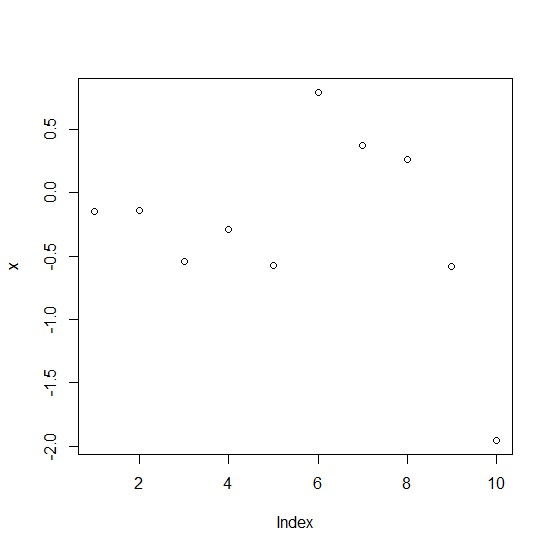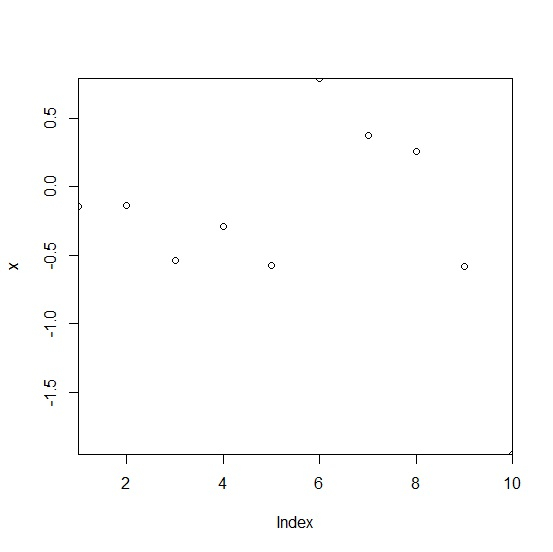# How to remove the plot margin in base R between the axes and the points inside the plot?

R ProgrammingServer Side ProgrammingProgramming

To remove the plot margin in base R between the axes and the points inside the plot, we can use xaxs and yaxs argument in plot function. Depending on the choices of the arguments xaxs and yaxs, the plot region in the respective direction is 4% larger than specified by these limits or exactly matches the "i" limits.

## Example

x<-rnorm(10)
plot(x)

## Output## Example

plot(x,xaxs="i",yaxs="i")

## Output